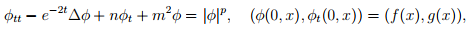### Special topics for classical damped wave models

Thời gian: 09:00 đến 11:30 ngày 22/11/2017, 09:00 đến 11:30 ngày 24/11/2017, 09:00 đến 11:30 ngày 30/11/2017,

Địa điểm: B4-705, VIASM

Báo cáo viên: Prof. M.Reissig, Faculty of Mathematics and Computer Science Technical University Bergakademie Freiberg, Germany

Tóm tắt:

Lecture Note.pdf

Schedule

1. 22.11.2017, 09.00-11.30: Phase space analysis for classical damped wave models

Content: First we derive representations of solutions by using Fourier multipliers. This allows to discuss decay behavior and decay rate of the wave type energy. Moreover, we explain the influence of additional regularity in the data. One of the most important properties is described by the so-called diffusion phenomenon for damped wave models. We explain this phenomenon. Some conclusions complete the first part of lectures.

2. 24.11.2017, 09.00-11.30: Semilinear classical damped wave models

Content: We begin with explanations of the Fujita exponent as the critical exponent in semilinear heat models. Then we show that this exponent is critical in semilinear classical damped wave models with power nonlinearity, too. Stability of the zero solution is proved by using decay estimates for solutions to parameter-dependent Cauchy problems, Duhamel’s principle and a fixed point argument. The optimality of the critical exponent is proved by applying the test function method. This implies blow-up (in finite time) of weak solutions even for small data.

3. 30.11.2017, 09.00-11.30: Fujita via Strauss - a never ending story

Content: First we recall the story of the Strauss exponent appearing as the critical exponent in semilinear wave models. We introduce Kato’s lemma as an important tool to prove blow-up results. Finally, we introduce recent results for some semilinear wave models with power nonlinearity and with scale invariant mass and dissipation. These models are taken from a grey zone, where an interaction of Fujita and Strauss critical exponents appears.

4. Lecture at HNUE: 29.11.2017 Title: Stability for semilinear de Sitter models with power nonlinearity Abstract: In this talk we consider the Cauchy problem for semilinear de Sitter models with power non-linearity. The model of interest iswhere m^2 is a nonnegative constant and n is the dimension. We study the global (in time) existence of small data solutions. In particular, we show the interplay between the power p, the regularity parameter of data spaces defined as special fractional Sobolev spaces and admissible spaces of solutions (in weak sense, in sense of energy solutions or in classical sense).